# Zoom Math 200 v3.04

## Zoom Math 200 Makes Algebra 1 Easy

Without Zoom Math 200, your calculator can’t do much algebra. But look at what Zoom 200 can do!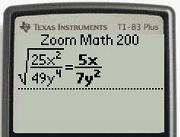Simplify a Square Root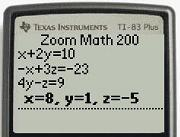Solving a System of Equations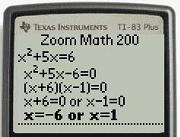Solving a Quadratic Equation Step by Step!

Even if you do not buy a Registration Key, Zoom Math 200 can solve some beginning algebra problems, including:

• Evaluating Variable Expressions
• Exponents and Powers
• Combining Like Terms
• The Distributive Property and FOIL
• Simplifying a Square Root

If you purchase a Registration Key, then Zoom Math 200 can solve most Algebra 1 problems, including:

• Linear Equations and Inequalities
• Systems of Linear Equations
• Factoring Polynomials
• Polynomial Division
• Sums of Arithmetic Sequences

Best of all, Zoom Math 200 can show the steps of most solutions, so you can see exactly how to do the math!

## Zoom Math 200 Makes Graphing Easy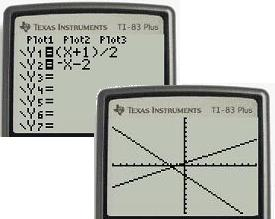Without Zoom Math 200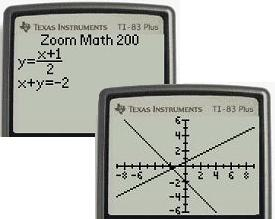With Zoom Math 200

Without Zoom Math, graphing can be confusing. If you want to see where the line $x+y=-2$ crosses the $x$-axis, you have to solve the equation for $y$, then type the equation in the special “Y=” screen, and then use the WINDOW menu or the ZOOM menu to find the spot you are looking for. And the graph is not labeled anyway!

Even if you do not buy a Registration Key, Zoom Math 200 makes it easy to graph equations that are very simple. To see where the line $x+y=-2$ crosses the $x$-axis, just type the equation, press GRAPH, and use the arrow keys to find the spot you are looking for. The graph is labeled, so you can easily read where the line crosses the $x$-axis.

If you purchase a Registration Key, then Zoom Math 200 can graph more equations.

## Zoom Math 200 Makes Calculations Easy

Even if you do not buy a Registration Key, Zoom Math 200 makes calculations easy. With Zoom Math installed on your graphing calculator, fractions look like fractions. Exponents look like exponents. Everything looks the way it does in your textbook. And you can quickly change your answer from decimal to fraction, mixed fraction, percentage, scientific notation, or quotient with remainder.

If you purchase a Registration Key, Zoom Math 200 can show you calculations one step at a time!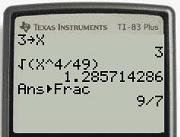Without Zoom Math 200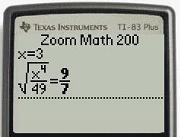Zoom Math 200(Unregistered)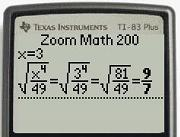Zoom Math 200(Registered)

## Quote

"Where I was spending 20+ hours on homework, Zoom reduced it to under 10." - Lori B. Los Angeles, CA

"The Trace function is invaluable because it allows me to relax and check my work. I can catch my errors on the spot." - Lori B., Los Angeles, CA

"What I like is that it allows me to totally grasp the concepts of Algebra like never before." Lori B, - Los Angeles, CA

"Prior to using Zoom I had debilitating anxiety over math success, now that fear is completely gone." - Lori B., Los Angeles, CA

"I am a 43 yr old mom of five sons and going back to college has been a struggle and math is one of my weakest areas. I must say that ZOOM MATH got me through this hard process wonderfully" - Sabrina K. Tampa, FL

"This app has saved my life!" - Natalie W. Portland, OR

"Your programs were instrumental in helping me pass and more importantly, understand, the Algebra and Business/Finance math courses that I had to take to obtain my Associates degree." - Mark C. Buffalo, NY

"Prior to teaching myself with Zoom Math my ACT on the math section was 24 three times in a row. After studying the problems I missed with Zoom Math's Trace feature as my guide I was able to pull my math section of the ACT up to a 31. I now have a 30 composite score and a full ride to the University of Alabama and you guys are 100% responsible!" - Andrew T., Sacramento, CA

"We received my son's MCT scores from 10th grade (end of last year). He jumped 3 levels and scored highest in his class." - Kathy J. Austin, TX

"I completed my math course 6 1/2 weeks ahead of time. It would not have been possible without your ZOOM 300 SOFTWARE. If I had your product at the beginning of my 24 week course I would have completed this course within 2 weeks." - Bernard P., Los Angeles, CA